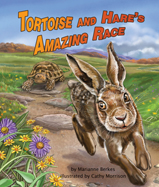Alignment to Standards for AK1 MEA-1. measuring and/or comparing objects using standard and nonstandard units (M2.1.2)
1 MEA-5. comparing concepts such as before/after, shorter/longer (M2.1.1)
2 MEA-1. measuring to the nearest inch or foot (M2.1.3)
2 MEA-2. comparing and ordering objects by length, weight, area, time, temperature (M2.1.1)
2 MEA-3. Compare objects to standard and nonstandard units to identify objects that are greater than, less than, and equal to a given unit (M2.1.2)
2 MEA-5. selecting and using appropriate tools of measurement (M2.1.3)
3 M2.1.2 Compare objects to standard and non-standard units to identify objects that are greater than, less than, and equal to a given unit.
3 M4.1.4 Complete open space sentences with missing numbers; use appropriate vocabulary including greater than, less than, and equal to; and use the correct symbols.
3 MEA-1 understanding of measurable attributes by estimating length to the nearest inch or foot (L) (M2.1.3)
3 MEA-2 compare and ordering objects according to measurable attribute (calendar, length, [temperature, weight, area, or volume L]) (M2.1.1)
3 MEA-3 understanding of measurable attributes by identifying or describing objects that are greater than, less than, or equal to a unit of measure (standard or non-standard) (M2.1.2)
3 MEA-6 ability to use measurement techniques using pictorial representations [or manipulatives L] in real-world contexts by measuring length to the nearest half-inch (M2.1.3)
4 M2.2.1 Estimate and measure weights, lengths, and temperatures to the nearest unit using the metric and standard systems.
4 MEA-2 understanding of measurable attributes by estimating temperature (degree Celsius or Fahrenheit) or weight (pounds or kilograms) to the nearest unit (L) (M2.2.1)
4 MEA-3 understanding of measurable attributes by identifying or using equivalent measures for length (inch, foot, yard: 12 inches = 1 foot, 3 feet = 1 yard, 36 inches = 1 yard; centimeter, meter: 100 centimeters = 1 meter) (M2.2.2)
4 MEA-4 understanding of measurable attributes by selecting an appropriate unit of metric measurement to estimate length, weight, or temperature (M2.2.1)
4 MEA-5 ability to use measurement techniques using pictorial representations [or manipulatives L] in real-world contexts by measuring length to the nearest half-inch or [centimeter L] (M2.2.1, M2.2.3, & M2.2.4)
4 SC2 understanding of the structure, function, behavior, development, life cycles, and diversity of living organisms.
5 M2.2.1 Estimate and measure weights, lengths, and temperatures to the nearest unit using the metric and standard systems.
5 M2.2.2 Identify and use equivalent measurements (e.g., 60 minutes = 1 hour, 7 days = 1 week).
5 MEA-1 understanding of measurable attributes by estimating length to the nearest one-fourth inch or centimeter (L) (M2.2.1)
5 MEA-2 understanding of measurable attributes by estimating temperature (degree Celsius or Fahrenheit, plus or minus 5 degrees) or weight (halfpounds or kilograms) to the nearest unit (L) (M2.2.1)
5 MEA-3 understanding of measurable attributes by identifying or using equivalent measures for weight/mass (16 oz. = 1 pound or 1000 grams = 1 kilogram), length (1000 millimeters = 1 meter), or time (M2.2.2)
5 MEA-4 ability to use measurement techniques by measuring temperature or weight using appropriate tools (L) (M2.2.1 & M2.2.3)
5 MEA-8 ability to use measurement techniques by measuring length to the nearest 1?4 inch or centimeter (M2.2.1)
K MEA-1. comparisons between objects using concepts of big/little, long/short, large/small, more/less, same (M2.1.1)
K MEA-3. identifying instruments used to measure length, time, and temperature (M2.1.3)

Back to Standards Page